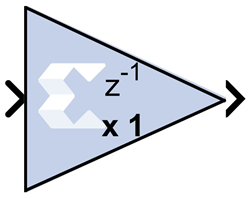# CMult - 2020.2 English

## Vivado Design Suite Reference Guide: Model-Based DSP Design Using System Generator (UG958)

Document ID
UG958
Release Date
2020-11-18
Version
2020.2 English

This block is listed in the following Xilinx® Blockset libraries: Math, Floating-Point, and Index.

The Xilinx CMult block implements a gain operator, with output equal to the product of its input by a constant value. This value can be a MATLAB® expression that evaluates to a constant.## Block Parameters

The block parameters dialog box can be invoked by double-clicking the icon in your Simulink® model.

Basic tab
Parameters specific to the Basic tab are as follows:
Constant Type
Fixed-point
Use fixed-point data type.
Floating-point
Use floating-point data type. Can be a constant or an expression. If the constant cannot be expressed exactly in the specified fixed-point type, its value is rounded and saturated as needed.
Fixed-point Precision
Number of bits
Specifies the bit location of the binary point of the constant, where bit zero is the least significant bit.
Binary point
Position of the binary point.
Floating-point Precision
Single
Specifies single precision (32 bits)
Double
Specifies double precision (64 bits)
Custom
Activates the field below so you can specify the Exponent width and the Fraction width.
Exponent width
Specify the exponent width.
Fraction width
Specify the fraction width.
Output tab
Precision
This parameter allows you to specify the output precision for fixed-point arithmetic. Floating point arithmetic output will always be Full precision.
Full
The block uses sufficient precision to represent the result without error.
User Defined
If you do not need full precision, this option allows you to specify a reduced number of total bits and/or fractional bits.
Fixed-point Output Type
Arithmetic type
Signed (2’s comp)
The output is a Signed (2’s complement) number.
Unsigned
The output is an Unsigned number.
Fixed-point Precision
Number of bits
Specifies the bit location of the binary point of the output number, where bit zero is the least significant bit.
Binary point
Position of the binary point in the fixed-point output.
Quantization

Refer to the section Overflow and Quantization.

Overflow

Refer to the section Overflow and Quantization.

Implementation tab
Parameters specific to the Implementation tab are as follows.
Use behavioral HDL description (otherwise use core)
When selected, System Generator uses behavioral HDL, otherwise it uses the Xilinx LogiCORE™ ™ Multiplier. When this option is not selected (false) System Generator internally uses the behavioral HDL model for simulation if any of the following conditions are true:
• The constant value is 0 (or is truncated to 0).
• The constant value is less than 0 and its bit width is 1.
• The bit width of the constant or the input is less than 1 or is greater than 64.
• The bit width of the input data is 1 and its data type is xlFix.
Core Parameters
Implement using
Specifies whether to use distributed RAM or block RAM.
Test for optimum pipelining
Checks if the Latency provided is at least equal to the optimum pipeline length supported for the given configuration of the block. Latency values that pass this test imply that the core produced is optimized for speed.

Other parameters used by this block are explained in the topic Common Options in Block Parameter Dialog Boxes.